#### You may also like### I'm Eight

Find a great variety of ways of asking questions which make 8.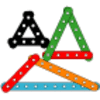### Let's Investigate Triangles

Vincent and Tara are making triangles with the class construction set. They have a pile of strips of different lengths. How many different triangles can they make?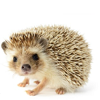### Noah

Noah saw 12 legs walk by into the Ark. How many creatures did he see?

# Two Numbers Under the Microscope

## Two Numbers Under the Microscope

Choose any two odd numbers, such as $5$ and $9$. Add them together.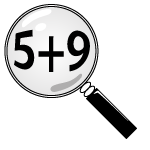Draw a picture or make a model to show how the numbers add together.

Adam found some dominoes with $5$ and $9$ spots on them: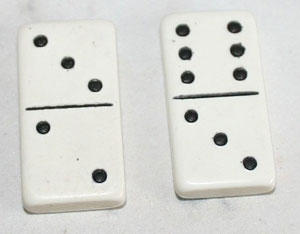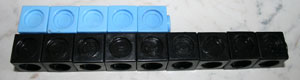Abdul drew a picture of $5$ add $9$ like this: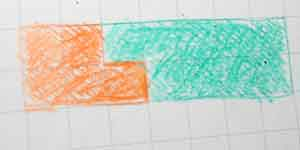Look closely at the models and pictures.

Can you see anything in any of them that would work in exactly the same way if you used two different odd numbers?

Can you use your one example to prove what will happen every time you add any two odd numbers?

See if you can explain this to someone else. Are they convinced by your argument?

Once you can convince someone else, see if you can find a way to show your argument. You might draw something or take a photo of things you have used to prove that your result is always true from your example.

### Why do this problem?

This problem supports the development of the idea of generic proof with the children. This is a tricky concept to grasp but it draws attention to mathematical structures that are not often addressed at primary school level. It is possible that only very few children in the class may grasp the idea but this is still a worthwhile activity which provides opportunities for children to explore odd and even numbers and the relationship between them. Proof is a fundamental idea in mathematics and in encouraging them to do this problem you will be helping them to behave like mathematicians.

By addressing the case of adding two odd numbers, a generic proof that adding two odd numbers always gives an even answer is developed based on the structure of odd and even numbers. The article entitled Take One Example will help you understand how this problem supports the development of the idea of generic proof with the children. Reading it will help you to see what is involved.

### Possible approach

Ask the children to choose two odd numbers and add them together. It is probably easiest if they choose ones that are easy to model and numbers that they are secure with.

Suggest that they make a model of their numbers using apparatus that is widely available in the classroom. Resist pointing them in specific directions unless they become stuck, but if they are then resources such as Multilink cubes, Numicon or squared paper will be helpful. After some time exploring they may need some prompting to move them towards looking at the pairing of their dots or cubes. These pictures may help.

The idea is that they take a particular example and then see if they can see the general structure within that one example.

### Key questions

How would you like to show these numbers?

Can you see anything in your example that would work in exactly the same way if you used two different odd numbers?

Can you say what will happen every time you add any two odd numbers?

Can you convince your friend that this is true?

### Possible extension

##### Even plus even

See what happens if you add two even numbers such as $4$ and $12$.
Can you 'see' in this example what will happen every time you add two even numbers?
You may find it helpful to work in a similar way to the way you worked for odd numbers.

##### Adding an odd and an even.

See what happens if you add an odd and an even or an even and an odd such as $6$ and $9$.
Can you 'see' in this example what will happen every time you add an odd and an even or an even and an odd number?

You may find it helpful to work in a similar way to the way you worked before.

You could go on to look at Take Three Numbers.

### Possible support

It may be helpful to encourage children to use paper cut outs of the numbers such as  these. In these the oddness of the odd numbers is very clear.  Laminated sets of these cards could be a very useful addition to your classroom resources. Numicon would be an alternative resource to use.